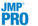Publication date: 07/30/2020

##Model Comparison Report

The Model Comparison Report contains the following information:

Show

A check box that indicates whether the Support Vector Machine Model report for the corresponding fit should appear in the report window.

Method

The model number.

Kernel Function

The kernel function for the corresponding model.

Cost

The value of the cost parameter for the corresponding model.

Gamma

The value of the gamma parameter for the corresponding model. If the model uses a linear kernel, this value is missing.

# SV

The number of support vectors used in the corresponding model.

Training Misclassification Rate

The misclassification rate for the observations in the training set. This rate is based on the classification decision rule that is calculated by the platform.

Validation Misclassification Rate

(Appears only if validation is used.) The misclassification rate for the observations in the validation set. This rate is based on the classification decision rule that is calculated by the platform.

Test Misclassification Rate

(Appears only if a test set is specified using a validation column.) The misclassification rate for the observations in the test set. This rate is based on the classification decision rule that is calculated by the platform.

Validation Generalized RSquare

(Appears only if validation is used.) The Generalized RSquare value for observations in the validation set. See Fit Details.

Probability Threshold

(Appears only if response is binary and validation is used.) The cutoff probability determined by the classification decision rule that is calculated by the platform. An observation is classified into the target level when its predicted probability exceeds this value.

Tip: You can change this value by using the Set Probability Threshold option in the Confusion Matrix report. See Confusion Matrix.

Conditional Validation Misclassification Rate

(Appears only if response is binary and validation is used.) The misclassification rate for the observations in the validation set, conditioned on the probability threshold value.

Best

Indicates which model fit has the smallest misclassification rate. If validation is used without a test set, this is the model with the smallest validation misclassification rate. If validation is used with a test set, this is the model with the smallest test misclassification rate.